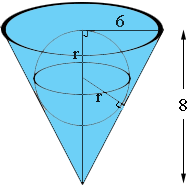SEARCH HOMEMath Central Quandaries & QueriesQuestion from Shubham, a student: Q. An upturned conical vessel of radius 6cm and height 8cm is completely filled with water. A sphere is lowered in the conical vessel filled with water and the size of sphere is such that it just touches the sides of cone and is just immersed. What fraction of water overflows?Shubham,

The volume of a cone of radius r and height h is 1/3 π r2 h. The volume of a sphere of radius r is 4/3 π r3. Since the sphere is completely immersed in the water the fraction of the water spilled is the volume of the sphere divided by the volume of the cone. You know the radius and height of the cone so all you need is the radius of the sphere.Maybe similar triangles will help. You may need to consult Pythagoras also.

HarleyMath Central is supported by the University of Regina and The Pacific Institute for the Mathematical Sciences.